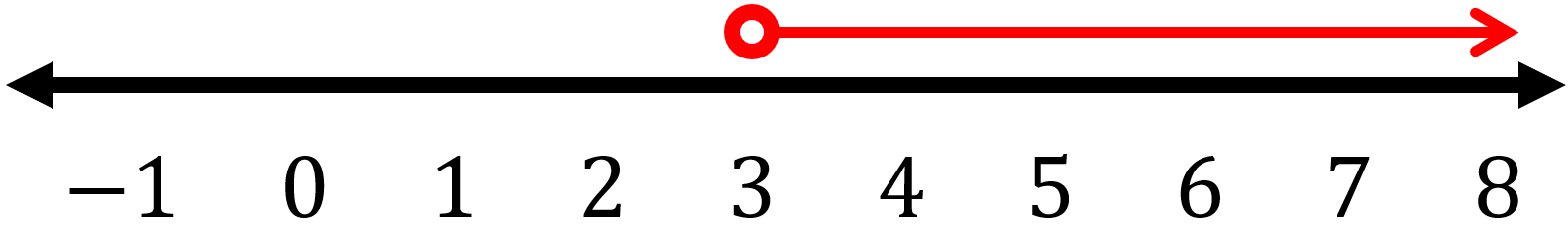## How to Solve Multi-Step Inequalities?

### Intro

Answering multi-step inequalities is nearly the same as solving equations. whatever is done to one side must be done to the other side so you can maintain the “balance” of the inequality. The Properties of Inequality can assist you in understanding how to add, subtract, multiply, or divide inside an inequality.

### Utilizing Properties Together for Solving Inequalities

A common technique used to solve equations, isolating a variable, additionally applies to answering inequalities. Via adding, subtracting, multiplying, and/or dividing, it’s possible to rewrite the inequality so the variable is on one side and all else is on the other. Like with one-step inequalities, the answers to multi-step inequalities may be graphed on a number line.

### Step-by-step guide for solving multi-step inequalities

• Isolate the variable which is similar to equations.
• Simplify utilizing the inverse of subtraction or addition.
• Simplify it further via utilizing the inverse of division or multiplication.
• In order to divide or multiply both sides using negative numbers, you must flip the direction of the inequality sign.

Example: Solve $$7x \ - \ 5 \ - \ 3x \ > \ 7$$

Firstly, one must isolate the variable term. The inverse operation of subtraction is addition. Therefore, add $$5$$ to both of the sides. $$7x \ - \ 5 \ - \ 3x \ > \ 7 \ ⇒ \ 7x \ - \ 5 \ - \ 3x \ + \ 5 \ > \ 7 \ + \ 5 \ ⇒ \ 7x \ - \ 3x \ > \ 12$$
Then, we must combine like terms: $$7x \ - \ 3x \ > \ 12 \ ⇒ \ 4x \ > \ 12$$
In order to isolate the variable $$x$$, divide both the sides by $$4$$. $$\frac{4x}{4} \ > \ \frac{12}{4} \ ⇒ \ x \ > \ 3$$

For graphing this inequality, one has to draw an open circle at the endpoint $$3$$ on the number line. This circle is open due to the fact the inequality is greater than $$3$$ and is not equal to $$3$$. The values where $$x$$ is greater than $$3$$ are seen all along the number line to the right of $$3$$. Draw a red line with an arrow on the number line that points in that direction.In order to check the answer, substitute the endpoint $$3$$ into the original inequality written as an equation. This is known as a related equation, to find out if it turns out to be a true statement. Afterward check another answer, such as $$0$$, to find out if the inequality is correct.

### Exercises for Multi Step Inequalities

1) $$4(x + 6) \ \geqslant \ 48$$$$\ \Rightarrow \$$

2) $$\frac{x - 12}{4} \ \geqslant \ -3$$$$\ \Rightarrow \$$

3) $$\frac{x - 12}{2} \ \geqslant \ -6$$$$\ \Rightarrow \$$

4) $$8(x + 19) \ \leqslant \ 176$$$$\ \Rightarrow \$$

5) $$4(x + 4) \ \leqslant \ 32$$$$\ \Rightarrow \$$

6) $$3(x + 2) \ \lt \ 27$$$$\ \Rightarrow \$$

7) $$7(x + 17) \ \lt \ 140$$$$\ \Rightarrow \$$

8) $$3(x + 3) \ \lt \ 33$$$$\ \Rightarrow \$$

9) $$6(x + 10) \ \lt \ 108$$$$\ \Rightarrow \$$

10) $$4(x + 12) \ \leqslant \ 52$$$$\ \Rightarrow \$$

1) $$4(x + 6) \ \geqslant \ 48$$$$\ \Rightarrow \ \color{red}{x + 6 \ \geqslant \frac{48}{4}}$$$$\ \Rightarrow \ \color{red}{x \ \geqslant \ 12 \ - \ 6}$$$$\ \Rightarrow \ \color{red}{x \ \geqslant \ 6}$$
2) $$\frac{x - 12}{4} \ \geqslant \ -3$$$$\ \Rightarrow \ \color{red}{x - 12 \ \geqslant -3 \times 4}$$$$\ \Rightarrow \ \color{red}{x \ \geqslant \ -12 \ + \ 12}$$$$\ \Rightarrow \ \color{red}{x \ \geqslant \ 0}$$
3) $$\frac{x - 12}{2} \ \geqslant \ -6$$$$\ \Rightarrow \ \color{red}{x - 12 \ \geqslant -6 \times 2}$$$$\ \Rightarrow \ \color{red}{x \ \geqslant \ -12 \ + \ 12}$$$$\ \Rightarrow \ \color{red}{x \ \geqslant \ 0}$$
4) $$8(x + 19) \ \leqslant \ 176$$$$\ \Rightarrow \ \color{red}{x + 19 \ \leqslant \frac{176}{8}}$$$$\ \Rightarrow \ \color{red}{x \ \leqslant \ 22 \ - \ 19}$$$$\ \Rightarrow \ \color{red}{x \ \leqslant \ 3}$$
5) $$4(x + 4) \ \leqslant \ 32$$$$\ \Rightarrow \ \color{red}{x + 4 \ \leqslant \frac{32}{4}}$$$$\ \Rightarrow \ \color{red}{x \ \leqslant \ 8 \ - \ 4}$$$$\ \Rightarrow \ \color{red}{x \ \leqslant \ 4}$$
6) $$3(x + 2) \ \lt \ 27$$$$\ \Rightarrow \ \color{red}{x + 2 \ \lt \frac{27}{3}}$$$$\ \Rightarrow \ \color{red}{x \ \lt \ 9 \ - \ 2}$$$$\ \Rightarrow \ \color{red}{x \ \lt \ 7}$$
7) $$7(x + 17) \ \lt \ 140$$$$\ \Rightarrow \ \color{red}{x + 17 \ \lt \frac{140}{7}}$$$$\ \Rightarrow \ \color{red}{x \ \lt \ 20 \ - \ 17}$$$$\ \Rightarrow \ \color{red}{x \ \lt \ 3}$$
8) $$3(x + 3) \ \lt \ 33$$$$\ \Rightarrow \ \color{red}{x + 3 \ \lt \frac{33}{3}}$$$$\ \Rightarrow \ \color{red}{x \ \lt \ 11 \ - \ 3}$$$$\ \Rightarrow \ \color{red}{x \ \lt \ 8}$$
9) $$6(x + 10) \ \lt \ 108$$$$\ \Rightarrow \ \color{red}{x + 10 \ \lt \frac{108}{6}}$$$$\ \Rightarrow \ \color{red}{x \ \lt \ 18 \ - \ 10}$$$$\ \Rightarrow \ \color{red}{x \ \lt \ 8}$$
10) $$4(x + 12) \ \leqslant \ 52$$$$\ \Rightarrow \ \color{red}{x + 12 \ \leqslant \frac{52}{4}}$$$$\ \Rightarrow \ \color{red}{x \ \leqslant \ 13 \ - \ 12}$$$$\ \Rightarrow \ \color{red}{x \ \leqslant \ 1}$$

## Multi Step Inequalities Practice Quiz

### HiSET Math Full Study Guide

$25.99$13.99

### STAAR Grade 7 Math Prep 2020

$20.99$15.99

### SSAT Middle Level Math Comprehensive Prep Bundle

$89.99$49.99

### FTCE Math Practice Workbook 2022

$25.99$12.99